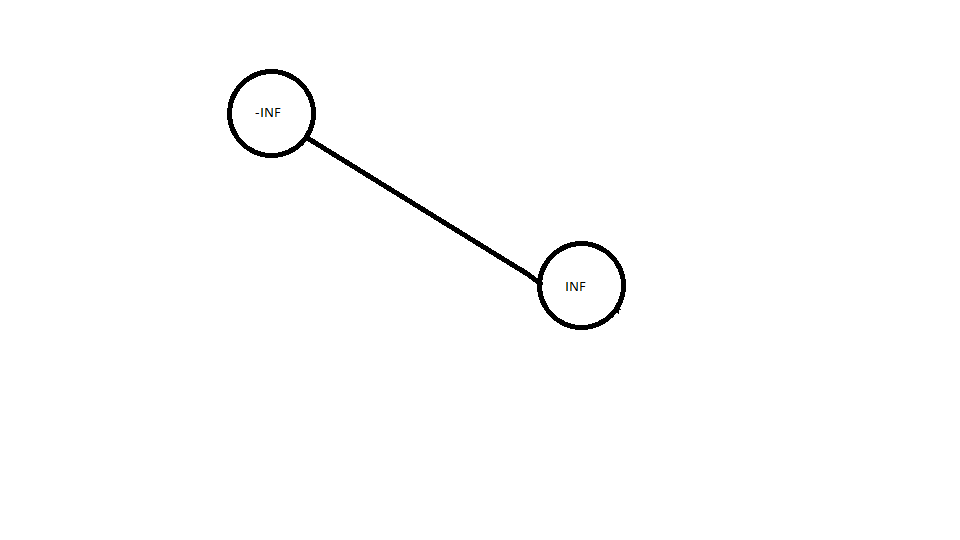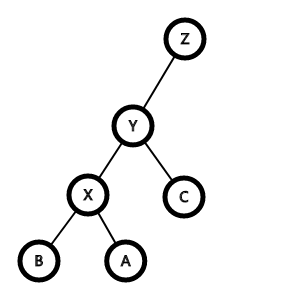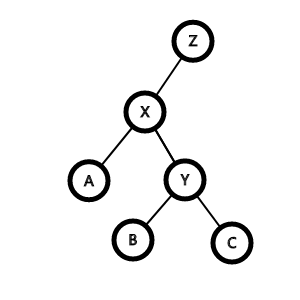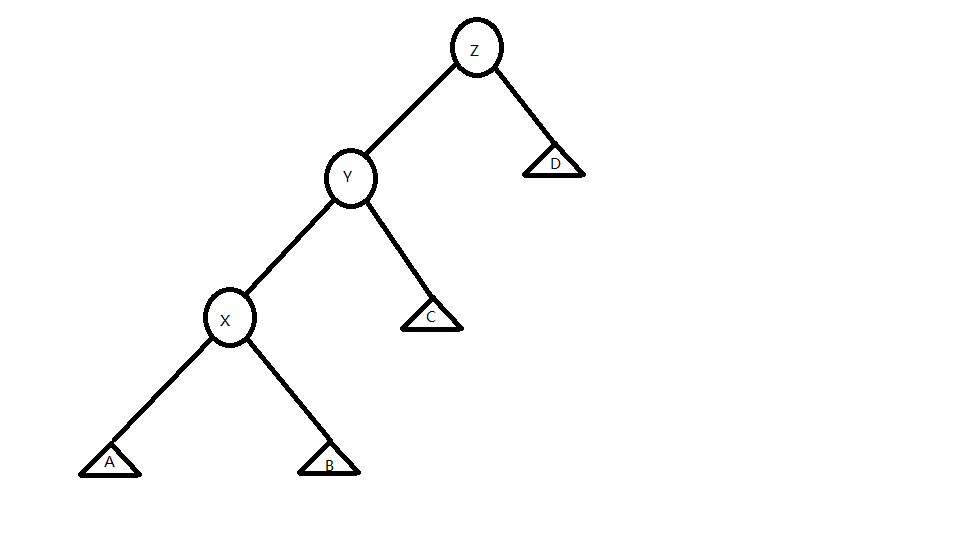# 正文：关于 SPLAY

• 该节点的关键码不小于它左子树的任意结点的关键码

• 该结点的关键码不大于它右子树的任意结点的关键码

显然，BST 的中序遍历是一个递增的序列

## 建立一棵 BSTconst int SIZE = 1e5 + 5;
const int INF = 0x7fffffff;
struct BSTNode {
int l, r; // 左右儿子的编号
int val; // 关键码
} T[SIZE];
int tot, root;

int clone(int val) { // 新建节点
T[++tot].val = val;
}

void build() {
clone(-INF), clone(INF);
root = 1, T.r = 2;
}


## SPLAY

splay，又称 cosplay 伸展树，有 “序列之王” 的美称，常数巨大，跑的没有 $fhq-treap$快，但这不在我们的讨论范围以内$$Y<Z, C>Y, X<Y,A<X,B>X$$

## 旋转

这个过程首先要满足 BST 性质$$odd\bigoplus1=odd-1$$

$$even\bigoplus1=even+1$$

即得易见平凡，仿照上例显然。

留作习题答案略，读者自证不难。

反之亦然同理，推论自然成立，略去过程 QED，由上可知证毕。

$Just$ $a$ $joke$

• $step1$: 将 Y 节点放到 X 节点的 w$\bigoplus$1 的位置
• $step2$: 如果 X 的 w$\bigoplus$1 位置上有一颗子树，放在 Y 的 w 位置上
inline void update(int x) { // 更新节点信息
T[x].siz = T[T[x].ch].siz + T[T[x].ch].siz + T[x].cnt;
}

inline void rotate(int x) { // 旋转
int y = T[x].fa; // X 它爹
int z = T[y].fa; // X 它爹它爹
int w = T[y].ch == x; // X 是它爹的左幺儿还是右幺儿
T[z].ch[T[z].ch == y] = x;
T[x].fa = z;
T[y].ch[w] = T[x].ch[w ^ 1];
T[T[x].ch[w ^ 1]].fa = y;
T[x].ch[w ^ 1] = y;
T[y].fa = x;
update(y), update(x);
}• $step1$: 如果三点共线，我们可以先旋转 X 它爹，这样便可以使其更加 “平衡”
• $step2$: 如果不共线……不共线……那就旋转 X 就好了

inline void splay(int x, int goal) { //splay
for (; T[x].fa ^ goal; rotate(x)) { // 一直旋转到 x 成为 goal 的儿子
int y = T[x].fa;
int z = T[y].fa;
if (z ^ goal)
T[y].ch ^ x ^ T[z].ch ^ y ? rotate(x) : rotate(y); //判断三点是否共线，如果是，就旋转 Y，否则旋转 X
}
if (!goal) root = x; // 把根节点设为 X
}



【模板】普通平衡树

• 题面：
您需要写一种数据结构（可参考题目标题），来维护一些数，其中需要提供以下操作：
1. 插入 x 数
2. 删除 x 数 (若有多个相同的数，因只删除一个)
3. 查询 x 数的排名 (排名定义为比当前数小的数的个数 +1 )
4. 查询排名为 x 的数
5. 求 x 的前驱 (前驱定义为小于 x，且最大的数)
6. 求 x 的后继 (后继定义为大于 x，且最小的数)


## 插入操作

inline void insert(int x) {
int u = root, fa = 0; // 从根节点开始找
while (u && x ^ T[u].val) // 找关键码相同的节点
fa = u, u = T[u].ch[x > T[u].val];
if (u) T[u].cnt++; // 如果有，就增加一个副本
else { // 否则新建一个节点
u = ++tot;
if (fa) T[fa].ch[x > T[fa].val] = u;
T[u].fa = fa;
T[u].siz = T[u].cnt = 1;
T[u].ch = T[u].ch = 0;
T[u].val = x;
}
splay(u, 0);
}


## 查找操作

inline void find(int x) {
int u = root;
if (!u) return ; // BST 空
while (T[u].ch[x > T[u].val] && x ^ T[u].val)
u = T[u].ch[x > T[u].val];
splay(u, 0);
}


## 前驱/后继操作

inline int next_bound(int x, int f) { // f=0 前驱，f=1 后继
find(x);
int u = root; // x 的父节点
if (T[u].val > x && f) return u;
if (T[u].val < x && !f) return u;
u = T[u].ch[f]; // 跳到对应的子树
while (T[u].ch[f ^ 1]) u = T[u].ch[f ^ 1]; // 反着跳转
return u;
}


## 删除操作

だから直接把 root 右幺儿的左幺儿删掉就可以了

inline void erase(int x) {
int last = next_bound(x, 0);
int next = next_bound(x, 1);
splay(last, 0), splay(next, last);
int del = T[next].ch;
if (T[del].cnt > 1) {
T[del].cnt--;
splay(del, 0);
}
else T[next].ch = 0;
}


## 第 K 大

inline int kth_element(int x) {
int u = root;
if (T[u].siz < x) return 0; // 没有那么多，直接死亡
while (233) {
int y = T[u].ch;
if (x > T[y].siz + T[u].cnt) {
x -= T[y].siz + T[u].cnt;
u = T[u].ch;
}
else if (T[y].siz >= x) u = y;
else return T[u].val;
}
}


#include <cstdio>
#include <iostream>
#include <algorithm>
#include <cstring>
#include <vector>

using namespace std;

const int SIZE = 1e5 + 5;
int n, root, tot;
struct SPLAY {
int fa;
int siz;
int cnt;
int val;
int ch;
} T[SIZE];

inline void update(int x) {
T[x].siz = T[T[x].ch].siz + T[T[x].ch].siz + T[x].cnt;
}

inline void rotate(int x) {
int y = T[x].fa;
int z = T[y].fa;
int w = T[y].ch == x;
T[z].ch[T[z].ch == y] = x;
T[x].fa = z;
T[y].ch[w] = T[x].ch[w ^ 1];
T[T[x].ch[w ^ 1]].fa = y;
T[x].ch[w ^ 1] = y;
T[y].fa = x;
update(y), update(x);
}

inline void splay(int x, int goal) {
for (; T[x].fa ^ goal; rotate(x)) {
int y = T[x].fa;
int z = T[y].fa;
if (z ^ goal)
T[y].ch ^ x ^ T[z].ch ^ y ? rotate(x) : rotate(y);
}
if (!goal) root = x;
}

inline void find(int x) {
int u = root;
if (!u) return ;
while (T[u].ch[x > T[u].val] && x ^ T[u].val)
u = T[u].ch[x > T[u].val];
splay(u, 0);
}

inline void insert(int x) {
int u = root, fa = 0;
while (u && x ^ T[u].val)
fa = u, u = T[u].ch[x > T[u].val];
if (u) T[u].cnt++;
else {
u = ++tot;
if (fa) T[fa].ch[x > T[fa].val] = u;
T[u].fa = fa;
T[u].siz = T[u].cnt = 1;
T[u].ch = T[u].ch = 0;
T[u].val = x;
}
splay(u, 0);
}

inline int next_bound(int x, int f) {
find(x);
int u = root;
if (T[u].val > x && f) return u;
if (T[u].val < x && !f) return u;
u = T[u].ch[f];
while (T[u].ch[f ^ 1]) u = T[u].ch[f ^ 1];
return u;
}

inline void erase(int x) {
int last = next_bound(x, 0);
int next = next_bound(x, 1);
splay(last, 0), splay(next, last);
int del = T[next].ch;
if (T[del].cnt > 1) {
T[del].cnt--;
splay(del, 0);
}
else T[next].ch = 0;
}

inline int kth_element(int x) {
int u = root;
if (T[u].siz < x) return 0;
while (233) {
int y = T[u].ch;
if (x > T[y].siz + T[u].cnt) {
x -= T[y].siz + T[u].cnt;
u = T[u].ch;
}
else if (T[y].siz >= x) u = y;
else return T[u].val;
}
}

signed main() {
scanf("%d", &n);
insert(1e9);
insert(-1e9);
for (int i = 1; i <= n; ++i) {
int opt, x;
scanf("%d %d", &opt, &x);
if (opt == 1) insert(x);
if (opt == 2) erase(x);
if (opt == 3) {
find(x);
printf("%d\n", T[T[root].ch].siz);
}
if (opt == 4) printf("%d\n", kth_element(x + 1));
if (opt == 5) printf("%d\n", T[next_bound(x, 0)].val);
if (opt == 6) printf("%d\n", T[next_bound(x, 1)].val);
}
return 0;
}


### 3 条评论#### ljx · 2020年1月20日 10:14 下午#### ljx · 2020年1月20日 10:13 下午

opt=3 时，应该要特判根节点的值是否小于 x